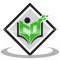## Can we say that the circle is a quadrilateral?Updated on 10-Oct-2022 09:47:44
Solution:No, we CANNOT say that a circle is a quadrilateral.A quadrilateral is a 2D closed plane figure made of 4 straight sides. The circle is a closed round curve. So a circle IS NOT a quadrilateral.

## Show $\\frac{3}{5}$ on a number line.Updated on 10-Oct-2022 09:47:43
Solution:Showing $\frac{3}{5}$ on the number lineAnswer $\frac{3}{5}$is a proper fraction. So it lies between 0 and 1 $\frac{3}{5}$ = 0.6. So it is shown as 0.6 on the Number Line as shown below

## You said real image is inverted, then why don't we see like that in cinema halls ?Updated on 10-Oct-2022 09:47:43
In cinema halls, they use some techniques to make sure that the final image is erect.The light from the reel is focused on a lens that forms a real inverted image and this light is then sent out from an opening which inverts the image further forming an erect image on the screen.

## Write abscissa and ordinate of point $(-3, -4)$Updated on 10-Oct-2022 09:47:43
Given: The coordinate is $(-3, -4).$ To find:  We have to find the abscissa and ordinate of the given coordinates. Solution: The abscissa OR x co-ordinate of the given point $(-3, -4) is -3$ The ordinate OR y co-ordinate of the given point $(-3, -4) is -4$

## Write the Co-ordinates of a point which lies on y-axis and is at a distance of 3 units above x-axis. Represent on the graph.Updated on 10-Oct-2022 09:47:42
The point lies on the y-axis means the x-coordinate is 0 and is at a distance of 3 units above the x-axis means its y coordinate is 3. So the point is (0, 3). The graph of (0, 3)

## Write the Co-ordinates of a point which lies on the x-axis and is at a distance of 4 units to the right of origin. Draw its graph.Updated on 10-Oct-2022 09:47:41
The point lies on X-axis, so is y coordinate is 0. It lies 4 units from origin, so its x-coordinate is 4. So the point is (4,0). The graph of (4,0)

## Write the name of each part of the plane formed by Vertical and horizontal lines.Updated on 10-Oct-2022 09:47:41
The vertical axis is the y-axis and its equation is x = 0 Vertical lines are given by the equation x = k where $k$ is an integer. The horizontal axis is the x-axis and its equation is y = 0 Horizontal lines are given by the equation y = k where k is an integer. The $x$-axis and the $y$-axis divides a plane into 4 quadrants, I, II, III, and IV in an anti-clockwise direction.

## Why Light(VIBGYOR) does not have white, brown or black colours?Updated on 10-Oct-2022 09:47:41
The black colour is just the absence of visible light.White colour is formed when all wavelengths of light are mixed. The other colours are due to different wavelengths of light. Many colours are formed by mixing different colours in VIBGYOR

## State whether True or FalseAll numbers which are divisible by 4 must also be divisible by 8.Updated on 10-Oct-2022 09:47:41
Answer:All numbers which are divisible by 4 must also be divisible by 8. FalseThe given statement is NOT true; for example, 20 is divisible by 4 but not divisible by 8.Test of divisibility for 4:Last two digits of given number must be divisible by 4Test of divisibility for 8:Last three digits of given number must be divisible by 8 All numbers which are divisible by 8 must also be divisible by 4. True. If a number is divisible by another number then it is divisible by each of the factors of that number. True

## Evaluate:1+2+3+.....+100 in a pattern.Updated on 10-Oct-2022 09:47:41
1 + 2 + 3....+ 100The above is the sum of the first 100 natural numbersAnswer:1 = 11 + 2 = 31 + 2 + 3 = 6 = 3(3+1)/21 + 2 + 3 + 4 = 10  = 4 (4+1)/2........From the above pattern, the formula has been developed as followsThe formula for the sum of the first n natural numbers is Sn = n(n + 1)/2 So the sum of the first 100 natural numbers, S100 = n(n + 1)/2 = 100(100+1)/2   = 50 x 101 = 5050